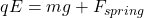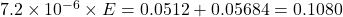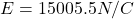## A spring with an unstrained length of 0.070 m and a spring constant of 1.9 N/m hangs vertically downward from the ceiling. A uniform electri

Question

A spring with an unstrained length of 0.070 m and a spring constant of 1.9 N/m hangs vertically downward from the ceiling. A uniform electric field directed upward fills the region containing the spring. A sphere with a mass of 5.8 × 10-3 kg and a net charge of +7.2 μC is attached to the lower end of the spring. The spring is released slowly, until it reaches equilibrium. The equilibrium length of the spring is 0.062 m. What is the magnitude of the external electric field?

in progress 0
7 months 2021-07-21T01:45:15+00:00 1 Answers 2 views 0

Electric field is equal to 15005.5 N/C

Explanation:

We have given unstrained length of spring = 0.070 m

Spring constant = 1.9 N/m

Equilibrium length of spring = 0.062 m

Change in length of spring x = 0.070 – 0.062 = 0.008 m

Mass of sphere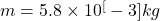Charge on sphere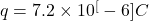Spring force is equal to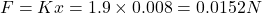Mg force on the sphere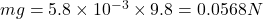Force on the sphere is equal to